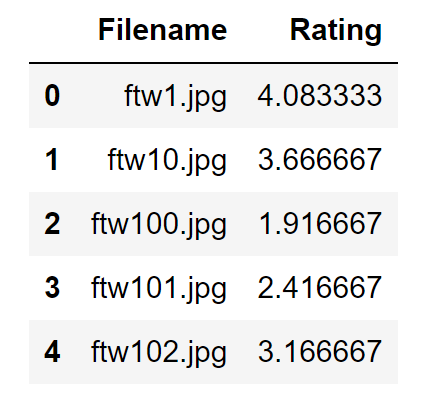# python数据分析处理库-Pandas基础操作

## 读取csv文件

``````import pandas as pd

print(numbers.dtypes)
``````

Filename object
Rating float64
dtype: object

# 读取数据

``````

numbers.tail(10)

numbers.columus
````````````
numbers.loc

numbers.loc[1:10]

numbers['Filename']
numbers['Filename','Rating']
``````

# 查找数据

``````n = numbers.columns.tolist()
m = []
for c in n:
if c.endswith('name'):
m.append(c)
print(m)
``````

['Filename']

`````` Filename
``````

0 ftw1.jpg
1 ftw10.jpg
2 ftw100.jpg
3 ftw101.jpg
4 ftw102.jpg

## 增加一列运算后的数据

``````total = numbers['placing_has_navi_no_mileage']+numbers['placing_has_navi_has_mileage']
numbers['total'] = total
``````

## 最大值、最小值、均值

``````print(numbers['total'].min())
print(numbers['total'].max())
print(numbers['total'].mean())
``````

## 排序

``````numbers.sort_values('total',inplace = True,ascending = False)
``````

## 筛选

``````
totalnull = pd.isnull(total)
totalnull.shape
print(totalnull)
numbers[:][totalnull == False]
``````

## 数据透视表

``````
p = numbers.pivot_table(index='order_id',values='total',aggfunc=np.mean)

numbers.dropna(axis=1)

numbers.dropna(axis=0,subset=['total','placing_has_navi_no_mileage'])
``````

Original: https://blog.csdn.net/Super_RD/article/details/123470615
Author: Super_RD
Title: python数据分析处理库-Pandas基础操作